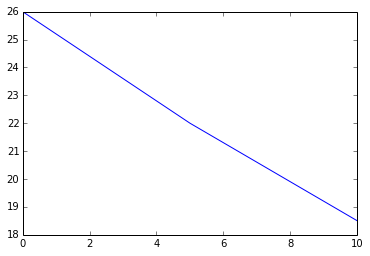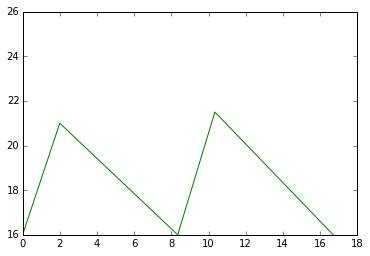# Chapter 16:Power Supplies¶

### Example 16.1 Page No 455¶

In :
#Given
#from fig. 16.1
Erms=24       #V, rms voltage

#Calculation
Em=1.4*Erms

#Result
print"The maximum instantaneous voltage is",round(Em,0),"V"

The maximum instantaneous voltage is 34.0 V


### Example 16.2 Page No 460¶

In :
#Given
#From fig 16.4 and 16.5
#at no load
Ilnl=0         #A, load current
Vdcnl=34	#V
Ilfl=1       #A, at full load
Vdcfl=24    #V
Il=0.5

#Calculation
Ro=(Vdcnl-Vdcfl)/(Ilfl)
Vdc=Vdcnl-Il*Ro

#result
print"Th output resistance is",Ro,"ohm"
print"The dc output voltage is",Vdc,"V"

Th output resistance is 10 ohm
The dc output voltage is 29.0 V


### Example 16.3 Page No 461¶

In :
#GIven
Vdcnl=34	#V, at no load
Ilfl=1          #A, at full load
Vdcfl=24        #V, at full load

#Calculation
regu=(Vdcnl-Vdcfl)*100/Vdcfl

#Result
print"Thepercent regulation is",round(regu,1),"percent"

Thepercent regulation is 41.0 percent


### Example 16.4 Page No 463¶

In :
#Given
If=1           #A, full load current
Vf=24          #V  full load voltage
C=1000 		#microF , capacitance

#Calculation
dvo=If/(200*C**10**-6)
Vrms=dvo/(3.5)
Vlmin=Vf-(round(dvo*1000,0)/2.0)

#result
print"(a)peak to peak and rms value of ripple voltage is",round(dvo*1000,0),"V and",round(Vrms*1000,2),"V"
print"(b) The minimum instanttaneous output voltage is",Vlmin,"V"

(a)peak to peak and rms value of ripple voltage is 5.0 V and 1.43 V
(b) The minimum instanttaneous output voltage is 21.5 V


### Example 16.5 Page No 463¶

In :
#Given
#from example 16.4
Vf=24.0           #V
Vrms=1.43        #V

#Calculation
ripple=Vrms/Vf*100

#result
print"The percentage ripple is",round(ripple,0),"percent"

The percentage ripple is 6.0 percent


### Example 16.6 Page No 464¶

In :
#Given
Vdcfl=12.0           #V, voltage
Ilfl=1.0             #A  current
ripp=10.0            #percent,  ripple
Ro=10.0              #ohm,  guess value

#calculation
#DC design procedure
#from  eq 16.3(a)
Vdcnl=Vdcfl+Ilfl*Ro
Em=Vdcnl
Erms=Em/1.4
#For a FWB rectifier
Isec=1.8*Ilfl
PIV=Vdcnl+(20/100.0)*Vdcnl

#AC design procedure
Vrms=(ripp/100.0)*Vdcfl
dvo=3.5*Vrms         #From eq 16.5 (b)
C=Ilfl/(200*dvo)
WVDC=Vdcnl+(20/100.0)*Vdcnl

#result
print" Transformer at :115V/18V at ",round(Isec,0),"A"
print"Four diodes :each 1 A at PIV",PIV,"V (or more)"
print"THree capacitors: 500 muF connected in parallel,WVDC=",WVDC,"V (or more)"

 Transformer at :115V/18V at  2.0 A
Four diodes :each 1 A at PIV 26.4 V (or more)
THree capacitors: 500 muF connected in parallel,WVDC= 26.4 V (or more)


### Analysis Example 16.7 Page No 466¶

In :
#Given
Ro=7		#ohm
Ilfl=1		#A,
C=1000          #microF

#Calculation
Erms=1.8*Vrms
Em=1.4*Erms
Vdcnl=Em
Vdcfl=Vdcnl-Ilfl*Ro
reg=(Vdcnl-Vdcfl)*100/Vdcfl

dvo=(Ilfl/200*C*10**-6)
Vrms=dvo/3.5
rip=Vrms*100/Vdcfl

#Result
print"(a)Vdcfl is",Vdcfl,"V and percentag eregulation is",round(reg,0)
print"(b)percentage ripple is",round(rip,1)

#Plot
I=[0,5,10]
VdcNL=[26,22,18.5]
a1=plot(I,VdcNL)
show(a1)

t1=[0,16.7]
Vdcnl=[26,26]
t2=[0,2,8.34,10.34,16.7]
Vdcfl=[16,21,16,21.5,16]
a2=plot(t1,Vdcnl)
a3=plot(t2,Vdcfl)
show(a2)
show(a3)

(a)Vdcfl is -7.0 V and percentag eregulation is -100.0
(b)percentage ripple is -0.0